GMAT Math : DSQ: Calculating median

Example Questions

2 Next →

Example Question #11 : Median

Given five distinct positive integers -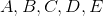- which of them is the median?

Statement 1: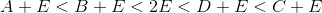Statement 1: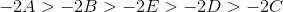BOTH STATEMENTS TOGETHER do NOT provide sufficient information to answer the question.

BOTH STATEMENTS TOGETHER provide sufficient information to answer the question, but NEITHER STATEMENT ALONE provides sufficient information to answer the question.

STATEMENT 1 ALONE provides sufficient information to answer the question, but STATEMENT 2 ALONE does NOT provide sufficient information to answer the question.

EITHER STATEMENT ALONE provides sufficient information to answer the question.

STATEMENT 2 ALONE provides sufficient information to answer the question, but STATEMENT 1 ALONE does NOT provide sufficient information to answer the question.

EITHER STATEMENT ALONE provides sufficient information to answer the question.

Explanation:

Assume Statement 1 alone. By the Addition Property of Inequality, if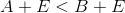,

then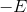can be added to each quantity to give an equivalent inequality: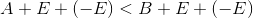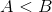.

By extension, the continued inequality in Statement 1 is equivalent to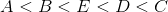.

A similar argument holds for Statement 2. By the Multiplication Property of Inequality, if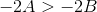, then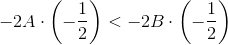andBy extension, the continued inequality in Statement 2 is also equivalent to.

The median of five numbers (an odd number) is the number in the middle when they are arranged in ascending order. Therefore, it can be established from either statement alone that of the five, the median is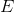.

Example Question #67 : Descriptive Statistics

Given five distinct positive integers -- which of them is the median?

Statement 1: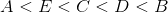Statement 2: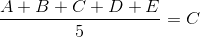EITHER STATEMENT ALONE provides sufficient information to answer the question.

BOTH STATEMENTS TOGETHER provide sufficient information to answer the question, but NEITHER STATEMENT ALONE provides sufficient information to answer the question.

STATEMENT 2 ALONE provides sufficient information to answer the question, but STATEMENT 1 ALONE does NOT provide sufficient information to answer the question.

BOTH STATEMENTS TOGETHER do NOT provide sufficient information to answer the question.

STATEMENT 1 ALONE provides sufficient information to answer the question, but STATEMENT 2 ALONE does NOT provide sufficient information to answer the question.

STATEMENT 1 ALONE provides sufficient information to answer the question, but STATEMENT 2 ALONE does NOT provide sufficient information to answer the question.

Explanation:

Assume Statement 1 alone. The median of five numbers (an odd number) is the number in the middle when they are arranged in ascending order. By Statement 1, this number is, so the question is answered.

Statement 2 alone, however, gives that the mean is. It is possible that the mean and the median can be one and the same or two different numbers.

Case 1: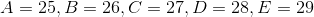The mean is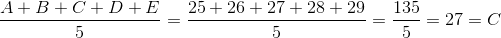making this consistent with Statement 2.

The median is the middle element,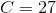.

Case 2: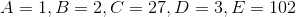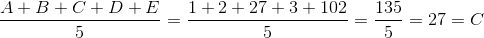again, making this consistent with Statement 2.

The median is the middle element,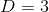.

Example Question #68 : Descriptive Statistics

What is the median of a data set comprising nineteen elements?

Statement 1: When arranged in ascending order, the ninth element is 72.

Statement 2: When arranged in descending order, the ninth element is 72.

Statement 1 ALONE is sufficient to answer the question, but Statement 2 ALONE is not sufficient to answer the question.

BOTH statements TOGETHER are insufficient to answer the question.

Statement 2 ALONE is sufficient to answer the question, but Statement 1 ALONE is not sufficient to answer the question.

EITHER statement ALONE is sufficient to answer the question.

BOTH statements TOGETHER are sufficient to answer the question, but NEITHER statement ALONE is sufficient to answer the question.

BOTH statements TOGETHER are sufficient to answer the question, but NEITHER statement ALONE is sufficient to answer the question.

Explanation:

In a data set of 19 elements, 19 being odd, the median is the element that occurs in the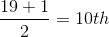position when they are arranged in ascending (or descending) order. Neither statement alone tells us what that middle element is. But put together, they tell us what the ninth and eleventh elements would be when arranged in ascending (or descending) order. Since both are 72, the element between them - the tenth element, and, thus, the median - must be 72.

The answer is that both statements together are sufficient to answer the question, but neither statement alone is sufficient to answer the question.

2 Next →

Tired of practice problems?

Try live online GMAT prep today.